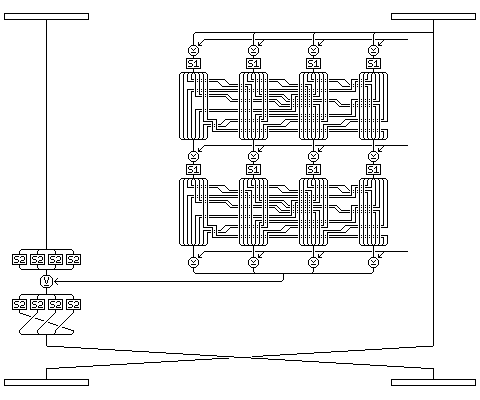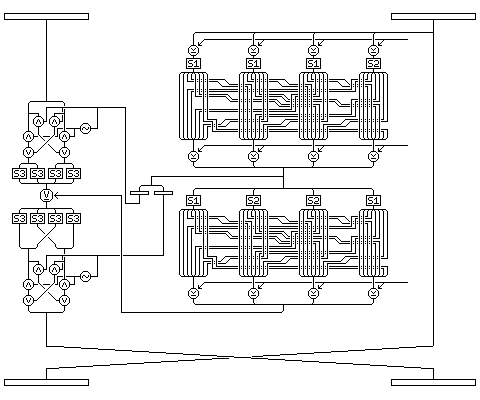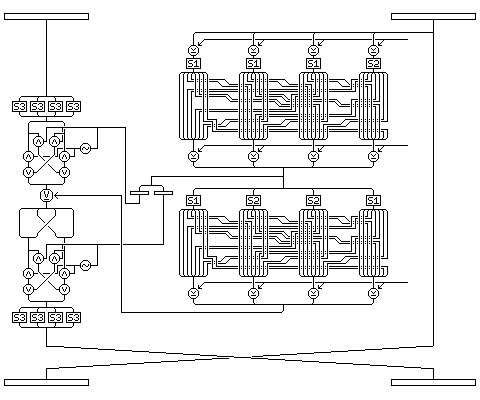[Next] [Up/Previous] [Index]

The intent of the following proposed block cipher is to provide a cipher which is at least as secure as DES, and possibly somewhat more secure, which is reasonably efficient when implemented in software, and is furthermore easy to implement.

 Changes required for QUADIBLOC 99, which corrects known weaknesses in the original QUADIBLOC (QUADIBLOC 96), are indicated in highlighted boxes.

Two S-boxes, taking 8 binary inputs uniquely to 8 binary outputs, are used, as well as the inverse of the second S-box. The S-boxes are constructed from Euler's constant (.57721...) as follows:

start with an array, A, such that A(0) is 0, A(1) is 1, up to A(255) which is 255. Place Euler's constant to sufficient precision in ACC. Number of choices starts at 256, and is decreased by 1 for each iteration; element to choose starts at 0, and increases by 1 for each iteration. The iteration where Number of choices is 2 is the last iteration.

During each iteration, multiply ACC by Number of choices. Leave the fractional part of the result in ACC; swap

``` A( Number of choices )
```

and

``` A( Number of choices + the integer part of the result).
```

This generates S-box 1; repeat the procedure with the contents remaining in ACC to obtain S-box 2. (ACC must be long enough to hold Euler's constant to sufficient precision to support both applications of the procedure.)

The S-boxes are given on the page entitled Euler's Constant and the Quadibloc S-boxes.

 In QUADIBLOC 99, a third S-box, generated by continuing the process, is also used.

In addition, the following 4 of 8 code for the numbers 0 to 63 is used during subkey generation:

given the 6 bits abcdef, in the output word, let c stand for 01 if the bit c is 0, or 10 if the bit c is 1, and let DD stand for 0011 if the bit d is 0, or 1100 if the bit d is 1, then

```00cdef becomes cdef
010def becomes DDef
011def becomes deFF
100def becomes dEEf
101def becomes DefD
110def becomes DeDf
111def becomes dEfE
```

or, giving the 64 equivalents in hex,

```55 56 59 5A 65 66 69 6A 95 96 99 9A A5 A6 A9 AA
35 36 39 3A C5 C6 C9 CA 53 5C 63 6C 93 9C A3 AC
4D 4E 71 72 8D 8E B1 B2 17 1B 27 2B D4 D8 E4 E8
1D 1E 2D 2E D1 D2 E1 E2 47 4B 74 78 87 8B B4 B8
```

The cipher is an iterative block cipher, with 16 rounds and 48 subkeys, and uses Feistel rounds: half the block is used to encipher the other half in each round. It operates on a 64-bit block, and has a 160-bit key.

Initially, the first 4 bytes of data to encrypt are taken as the left half, and the last 4 bytes are taken as the right half.

### Each round proceeds as follows:

A copy of the right half, which will actually be unchanged by this round, is taken.

### This now describes the f-function

The copy is XORed with the round's first subkey (subkey 1 for round 1, subkey 4 for round 2, to subkey 46 for round 16).

Then, each byte is replaced by its substitute in S-box 1.

 In QUADIBLOC 99, the fourth byte is instead replaced by its substitute in S-box 2.

The bits of the result, considered to be numbered from 1 (most significant bit of the first, leftmost byte) to 32 (least significant bit of the last, rightmost byte) following the pattern in DES, are to be transposed to lie in the following positions:

``` 1  2 27 28 21 22 15 16
9 10  3  4 29 30 23 24
17 18 11 12  5  6 31 32
25 26 19 20 13 14  7  8
```

Note that this arrangement posesses a great deal of symmetry: only ONE version of S-box 1, with 256 32-bit entries is needed to perform both the S-box substitution, and the subsequent permutation, in a single step for efficiency on a computer without hardware instructions for bit transposition. And, since no bits change their position within a byte, a slower implementation, using S-box 1 with single byte entries, and doing the transposition using masking, is also possible.

 In QUADIBLOC 99, the symmetry of the bit transposition remains, but one expanded version of S-box S2 is also required.

The 32 transposed bits are now XORed with the round's second subkey.

 In QUADIBLOC 99, the value generated at this point is also retained, and is called the intermediate result of the f-function.

Each byte of the result is again replaced by its substitute in S-box 1, and the bits of the result are transposed as before.

 In QUADIBLOC 99, in the second part of the f-function, the second and third bytes are instead replaced by their substitutes in S-box 2.

The result is XORed with the round's third subkey. This produces the output of the f-function.

### Applying the f-function output to alter the left half of the block:

 In a QUADIBLOC 99 type A round, the first (leftmost) half of the intermediate result of the f-function is used to control an ICE-style swap of bits between the halves of the left half of the block at this time: each bit in that 16-bit quantity which is 1 indicates that corresponding bits in the two halves of the 32-bit left half of the block are to be swapped.

Each byte of the left half is replaced by its substitute in S-box 2.

 In QUADIBLOC 99, S-box 3 is used for this purpose. In a QUADIBLOC 99 type B round, the first (leftmost) half of the intermediate result of the f-function is used to control an ICE-style swap of bits between the halves of the left half of the block at this time: each bit in that 16-bit quantity which is 1 indicates that corresponding bits in the two halves of the 32-bit left half of the block are to be swapped.

The result is XORed with the result of the f-function applied to the right half.

 In a QUADIBLOC 99 type B round, first the second and third bytes of the left half of the block are swapped, and then the second (rightmostmost) half of the intermediate result of the f-function is now used to control another ICE-style swap of bits between the halves of the left half of the block: each bit in that 16-bit quantity which is 1 indicates that corresponding bits in the two halves of the 32-bit left half of the block are to be swapped.

Each byte of the result is replaced by its substitute in S-box 2.

In QUADIBLOC 99, S-box 3 is used for this purpose.

In a QUADIBLOC 99 type A round, first the second and third bytes of the left half of the block are swapped, and then the second (rightmostmost) half of the intermediate result of the f-function is now used to control another ICE-style swap of bits between the halves of the left half of the block: each bit in that 16-bit quantity which is 1 indicates that corresponding bits in the two halves of the 32-bit left half of the block are to be swapped.

### The sequence of rounds:

In QUADIBLOC 99, the type A and type B rounds alternate as follows:

```Round:  1  2  3  4  5  6  7  8  9 10 11 12 13 14 15 16
Type:   A  A  B  B  A  A  B  B  B  B  A  A  B  B  A  A
```

Except for the four middle rounds, this ensures that the ICE-style bit transpositions alternate with the byte substitutions using S-box 3.

### The swap:

After every round except round 8 and round 16:

the left half for the next round is the unchanged right half from the previous round;

the right half for the next round is the modified left half, after the XOR and the two substitutions, subjected to a circular left shift of 8 bits (which can be carried out by moving whole bytes, of course).

 In QUADIBLOC 99, the swap is performed in all rounds except round 16 only. The bit transpose after round 8 is removed as not having as much effectiveness as was hoped for, and instead the ICE-style bit swap is added to every round. The circular left shift of 8 bits is no longer part of the swap, and is replaced by the exchange of two bytes preceding the last bit swap.

After round 8:

Each byte of the right half is replaced by its substitute in S-box 2.

Subject the bits of the block, numbered from 1 to 64, from left to right, to the following (reciprocal) bit transpose:

``` 1 34 11 44 21 54 31 64   9 42  3 36 29 62 23 56
17 50 27 60  5 38 15 48  25 58 19 52 13 46  7 40
33  2 43 12 53 22 63 32  41 10 35  4 61 30 55 24
49 18 59 28 32  6 47 16  57 26 51 20 45 14 39  8
```

Each byte of the right half of the result is replaced by its substitute in S-box 2.

 As previously mentioned, this operation is completely omitted from QUADIBLOC 99, and a simple swapping of halves takes place instead.

After round 16:

Nothing happens: the result at that point, without further swapping, is the output of the cipher.

The following diagram illustrates what happens during a normal round of QUADIBLOC 96 (from 1 to 7, or 9 to 15), to help make the description clearer:And these are the corresponding diagrams for QUADIBLOC 99: For a type A round:For a type B round:### Some comments at this stage:

Using a 'double' f-function means:

a) every bit of the f-function output depends on every bit of the right half of the block, thus making propagation very rapid, and

b) the first half of the f-function can be thought of as substituting for the absence of an expansion permutation and auxilliary S-box inputs as found in DES.

Decipherment is the same as encipherment, except:

a) S-box 2 is replaced by the inverse of S-box 2 (S-box 1 is unchanged),

 In QUADIBLOC 99, for decipherment, S-box 3 is replaced by its inverse, and S-boxes 1 and 2 are unchanged.

b) after every round except rounds 8 and 16, the modified left half from one round becomes the right half for the next, and the unmodified right half receives a right circular shift of 8 bits before becoming the left half for the next round, and

 In QUADIBLOC 99, the swap of the second and third bytes in the left half of the block is changed to take place after the first ICE-style swap and before the first use of S-box 3. Also, now the ICE-style swap that occurs first uses the second (rightmost) half of the intermediate result of the f-function as input, and the ICE-style swap that occurs second uses the first (leftmost) half of the intermediate result of the f-function as input.

c) the 16 groups of three subkeys for the 16 rounds are used in reverse order, but the three subkeys within each group are still used in the same order.

The bit transpose with partial substitution between rounds 8 and 9 is intended to create a 'wall' between the first 8 and the last 8 rounds that will make the cipher much harder to analyze and solve.

 The bit transpose has been removed since the boomerang attack has cast some doubts on its efficacy.

### Subkey generation:

The 160-bit key shall be expanded to 176 bits by applying the 4 of 8 bit code specified above to each group of 6 bits in the last 48 bits of the key, thus expanding these 48 bits to 64 bits. (This is done to prevent weak keys.)

 In QUADIBLOC 99, the last 64 bits of the 160-bit key shall be used, reduced to 48 bits by ignoring the most significant two bits of each byte, as input to the 4 of 8 bit code. This avoids having to perform unnecessary shift operations. Then, the 160-bit key will be expanded to the following: the first 96 bits of the original 160-bit key, the 64 bits generated from the 4 of 8 code, and the last 64 bits of the original 160-bit key XOR the first 64 bits of the original 160-bit key (so that the expanded key does not contain both the original form, and the 4 of 8 encoding, of the same bits) to produce an expanded key that is 224 bits long.

The first 128 bits of the result shall be divided into four 32-bit blocks, which shall be called, from left to right, P, Q, R, and S.

The subkeys shall be generated for the 16 encipherment rounds in order.

P, Q, and R will be taken as the three subkeys for the current round, subject to an XOR to be subsequently described.

Then, P, Q, and R shall be shifted left n bits, and S shall be shifted left 3n bits, with the first n bits of P, then the first n bits of Q, then the first n bits of R being shifted into S, while the bits shifted out of S into P, Q, and R will alternate, one bit at a time, into these three registers/locations.

Thus, when n is 6, we have:

```Before:

P) P1 P2 ... P32
Q) Q1 Q2 ... Q32
R) R1 R2 ... R32
S) S1 S2 ... S32

and after:

P) P7 P8 ... P32 S1 S4 S7 S10 S13 S16
Q) Q7 Q8 ... Q32 S2 S5 S8 S11 S14 S17
R) R7 R8 ... R32 S3 S6 S9 S12 S15 S18
S) S19 S20 ... S32 P1 P2 ... P6 Q1 Q2 ... Q6 R1 R2 ... R6
```

The value of n to use for each round in turn shall be

```5, 6, 5, 5,
7, 7, 7, 7,
7, 8, 7, 7,
5, 6, 5, 5.
```

Each of the 48 subkeys thus generated will now be XORed with the leftmost 32 bits of T, where T begins as the last 48 bits of the expanded key, and is given a right circular shift of 17 bits after each use.

 In QUADIBLOC 99, a right circular shift of 11 bits will be used after generating all but the last of the 27 subkeys used by the first nine rounds, and then a right circular shift of 17 bits will be used afterwards. Since the expanded key is 224 bits long, rather than 176 bits long, T will be 96 bits long and will initially contain the last 96 bits of the expanded key.

[Next] [Up/Previous] [Index]